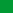Db2 11.5

# Grouping by SELECT clause columns

When operating in NPS compatibility mode, you can specify the ordinal position or exposed name of a SELECT clause column when grouping the results of a query.

A GROUP BY clause groups the results of a query that have matching values for one or more grouping expressions. Each column included in a grouping expression must unambiguously identify a column of the query's SELECT clause or an exposed column of the query's intermediate result. If a SELECT clause contains column expressions that are not aggregate expressions, and if a GROUP BY clause is specified, those column expressions must be in the GROUP BY clause. For example:
``````SELECT c1 as a, c2+c3 as b, COUNT(*) as c
FROM t1
GROUP BY c1, c2+c3;``````
The syntax of a GROUP BY clause is described in group-by-clause.
Whether NPS compatibility mode is being used depends on the setting of the SQL_COMPAT global variable:
• When SQL_COMPAT='NPS', a grouping expression can refer to a SELECT clause column not only by its name, but also by its ordinal position in the SELECT clause or by its exposed name. This applies to simple grouping expressions, grouping sets, and super groups. The following restrictions apply:
• An expression is not allowed on an ordinal position or exposed name of a SELECT clause.
• An integer expression is not treated as an ordinal position of a SELECT clause. Instead, it is treated as a constant and results are grouped by the constant value.
• The ordinal position of a SELECT clause column cannot be less than one or greater than the number of columns in the result.
• Otherwise, a grouping expression can refer to a SELECT clause column only by its name, not by its ordinal position in the SELECT clause or by its exposed name.

## Examples

The following examples illustrate the use of ordinal positions and exposed names of SELECT clause columns in GROUP BY clauses when SQL_COMPAT='NPS':
• A simple grouping expression in which columns c1 and c2+c3 are referred to by their ordinal positions in the SELECT clause (1 and 2):
``````SELECT c1 AS a, c2+c3 AS b, COUNT(*) AS c
FROM t1
GROUP BY 1, 2;``````
• A simple grouping expression in which columns c1 and c2+c3 are referred to by their exposed names (a and b):
``````SELECT c1 AS a, c2+c3 AS b, COUNT(*) AS c
FROM t1
GROUP BY a, b;``````
• A simple grouping expression in which the GROUP BY clause also references an exposed column of the query's intermediate result (c6):
``````SELECT c1 as a, c2+c3 as b, c4 || c5 as c, COUNT(*) as d
FROM t1
GROUP BY 1, b, c4, c5, c6;``````
• A grouping set in which columns c1 and c2+c3 are referred to by their ordinal positions in the SELECT clause (1 and 2):
``````SELECT c1 AS a, c2+c3 AS b, COUNT(*) AS c
FROM t1
GROUP BY GROUPING SETS ((1, 2), (1), (2));``````
• A grouping set in which columns c1 and c2+c3 are referred to by their exposed names (a and b):
``````SELECT c1 AS a, c2+c3 AS b, COUNT(*) AS c
FROM t1
GROUP BY GROUPING SETS ((a, b), (a), (b));``````
• A grouping set in which columns c1 and c2+c3 are referred to by both their ordinal positions in the SELECT clause (1 and 2) and their exposed names (a and b):
``````SELECT c1 AS a, c2+c3 AS b, COUNT(*) AS c
FROM t1
GROUP BY GROUPING SETS ((1, b), (a), (2));``````
• A super group in which columns c1 and c2+c3 are referred to by their ordinal positions in the SELECT clause (1 and 2):
``````SELECT c1 AS a, c2+c3 AS b, COUNT(*) AS c
FROM t1
GROUP BY ROLLUP (1), CUBE (1, 2);``````
• A super group in which columns c1 and c2+c3 are referred to by their exposed names (a and b):
``````SELECT c1 AS a, c2+c3 AS b, COUNT(*) AS c
FROM t1
GROUP BY ROLLUP (a), CUBE (a, b);``````
• A super group in which columns c1 and c2+c3 are referred to by both their ordinal positions in the SELECT clause (1 and 2) and their exposed names (a and b):
``````SELECT c1 AS a, c2+c3 AS b, COUNT(*) AS c
FROM t1
GROUP BY a, ROLLUP (a, 2), CUBE (b);``````
• Each of the following statements groups by the column t.c1:
``````SELECT c1 FROM t GROUP BY 1
SELECT c1 FROM t GROUP BY c1
SELECT c1 AS c1_alias FROM t GROUP BY c1_alias``````
However, in the following statement, the 1 in c2+1 is interpreted as a number, not as an ordinal position, so c2+1 is not equivalent to c2+c1:
``SELECT c1,c2 FROM t GROUP BY c2+1``